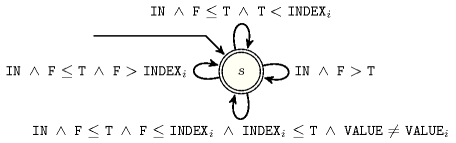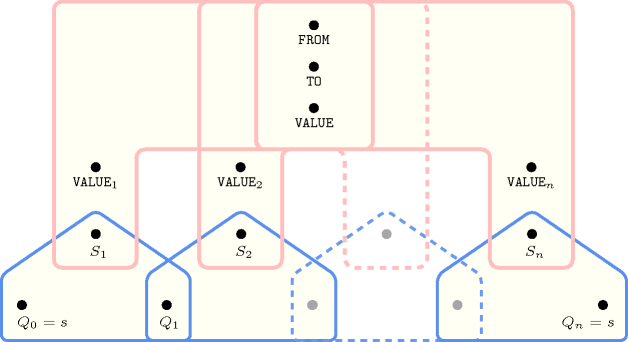## 5.138. elem_from_to

Origin

Derived from $\mathrm{𝚎𝚕𝚎𝚖}$.

Constraint

$\mathrm{𝚎𝚕𝚎𝚖}_\mathrm{𝚏𝚛𝚘𝚖}_\mathrm{𝚝𝚘}\left(\mathrm{𝙸𝚃𝙴𝙼},\mathrm{𝚃𝙰𝙱𝙻𝙴}\right)$

Synonym

$\mathrm{𝚎𝚕𝚎𝚖𝚎𝚗𝚝}_\mathrm{𝚏𝚛𝚘𝚖}_\mathrm{𝚝𝚘}$.

Arguments
 $\mathrm{𝙸𝚃𝙴𝙼}$ $\mathrm{𝚌𝚘𝚕𝚕𝚎𝚌𝚝𝚒𝚘𝚗}\left(\begin{array}{c}\mathrm{𝚏𝚛𝚘𝚖}-\mathrm{𝚍𝚟𝚊𝚛},\hfill \\ \mathrm{𝚌𝚜𝚝}_\mathrm{𝚏𝚛𝚘𝚖}-\mathrm{𝚒𝚗𝚝},\hfill \\ \mathrm{𝚝𝚘}-\mathrm{𝚍𝚟𝚊𝚛},\hfill \\ \mathrm{𝚌𝚜𝚝}_\mathrm{𝚝𝚘}-\mathrm{𝚒𝚗𝚝},\hfill \\ \mathrm{𝚟𝚊𝚕𝚞𝚎}-\mathrm{𝚍𝚟𝚊𝚛}\hfill \end{array}\right)$ $\mathrm{𝚃𝙰𝙱𝙻𝙴}$ $\mathrm{𝚌𝚘𝚕𝚕𝚎𝚌𝚝𝚒𝚘𝚗}\left(\mathrm{𝚒𝚗𝚍𝚎𝚡}-\mathrm{𝚒𝚗𝚝},\mathrm{𝚟𝚊𝚕𝚞𝚎}-\mathrm{𝚍𝚟𝚊𝚛}\right)$
Restrictions
 $\mathrm{𝚛𝚎𝚚𝚞𝚒𝚛𝚎𝚍}$$\left(\mathrm{𝙸𝚃𝙴𝙼},\left[\mathrm{𝚏𝚛𝚘𝚖},\mathrm{𝚌𝚜𝚝}_\mathrm{𝚏𝚛𝚘𝚖},\mathrm{𝚝𝚘},\mathrm{𝚌𝚜𝚝}_\mathrm{𝚝𝚘},\mathrm{𝚟𝚊𝚕𝚞𝚎}\right]\right)$ $\mathrm{𝙸𝚃𝙴𝙼}.\mathrm{𝚏𝚛𝚘𝚖}\ge 1$ $\mathrm{𝙸𝚃𝙴𝙼}.\mathrm{𝚏𝚛𝚘𝚖}\le |\mathrm{𝚃𝙰𝙱𝙻𝙴}|$ $\mathrm{𝙸𝚃𝙴𝙼}.\mathrm{𝚝𝚘}\ge 1$ $\mathrm{𝙸𝚃𝙴𝙼}.\mathrm{𝚝𝚘}\le |\mathrm{𝚃𝙰𝙱𝙻𝙴}|$ $\mathrm{𝙸𝚃𝙴𝙼}.\mathrm{𝚏𝚛𝚘𝚖}\le \mathrm{𝙸𝚃𝙴𝙼}.\mathrm{𝚝𝚘}$ $|\mathrm{𝙸𝚃𝙴𝙼}|=1$ $\mathrm{𝚛𝚎𝚚𝚞𝚒𝚛𝚎𝚍}$$\left(\mathrm{𝚃𝙰𝙱𝙻𝙴},\left[\mathrm{𝚒𝚗𝚍𝚎𝚡},\mathrm{𝚟𝚊𝚕𝚞𝚎}\right]\right)$ $\mathrm{𝚃𝙰𝙱𝙻𝙴}.\mathrm{𝚒𝚗𝚍𝚎𝚡}\ge 1$ $\mathrm{𝚃𝙰𝙱𝙻𝙴}.\mathrm{𝚒𝚗𝚍𝚎𝚡}\le |\mathrm{𝚃𝙰𝙱𝙻𝙴}|$ $\mathrm{𝚒𝚗𝚌𝚛𝚎𝚊𝚜𝚒𝚗𝚐}_\mathrm{𝚜𝚎𝚚}$$\left(\mathrm{𝚃𝙰𝙱𝙻𝙴},\left[\mathrm{𝚒𝚗𝚍𝚎𝚡}\right]\right)$
Purpose

Let $\mathrm{𝙵𝚁𝙾𝙼}$, $\mathrm{𝙲𝚂𝚃}_\mathrm{𝙵𝚁𝙾𝙼}$, $\mathrm{𝚃𝙾}$, $\mathrm{𝙲𝚂𝚃}_\mathrm{𝚃𝙾}$, $\mathrm{𝚅𝙰𝙻𝚄𝙴}$ respectively denote the attributes $\mathrm{𝙸𝚃𝙴𝙼}\left[1\right].\mathrm{𝚏𝚛𝚘𝚖}$, $\mathrm{𝙸𝚃𝙴𝙼}\left[1\right].\mathrm{𝚌𝚜𝚝}_\mathrm{𝚏𝚛𝚘𝚖}$, $\mathrm{𝙸𝚃𝙴𝙼}\left[1\right].\mathrm{𝚝𝚘}$, $\mathrm{𝙸𝚃𝙴𝙼}\left[1\right].\mathrm{𝚌𝚜𝚝}_\mathrm{𝚝𝚘}$, $\mathrm{𝙸𝚃𝙴𝙼}\left[1\right].\mathrm{𝚟𝚊𝚕𝚞𝚎}$ of the unique item of the $\mathrm{𝙸𝚃𝙴𝙼}$ collection.

Beside imposing the fact that $\mathrm{𝙵𝚁𝙾𝙼}\le \mathrm{𝚃𝙾}$ and that both $\mathrm{𝙵𝚁𝙾𝙼}$ and $\mathrm{𝚃𝙾}$ are assigned a value in $\left[1,|\mathrm{𝚃𝙰𝙱𝙻𝙴}|\right]$, the $\mathrm{𝚎𝚕𝚎𝚖}_\mathrm{𝚏𝚛𝚘𝚖}_\mathrm{𝚝𝚘}$ constraint forces the following condition: All entries of the $\mathrm{𝚃𝙰𝙱𝙻𝙴}$ collection from position $max\left(1,\mathrm{𝙵𝚁𝙾𝙼}+\mathrm{𝙲𝚂𝚃}_\mathrm{𝙵𝚁𝙾𝙼}\right)$ to position $min\left(|\mathrm{𝚃𝙰𝙱𝙻𝙴}|,\mathrm{𝚃𝙾}+\mathrm{𝙲𝚂𝚃}_\mathrm{𝚃𝙾}\right)$ are equal to $\mathrm{𝚅𝙰𝙻𝚄𝙴}$. When $max\left(1,\mathrm{𝙵𝚁𝙾𝙼}+\mathrm{𝙲𝚂𝚃}_\mathrm{𝙵𝚁𝙾𝙼}\right)$ is strictly greater than $min\left(|\mathrm{𝚃𝙰𝙱𝙻𝙴}|,\mathrm{𝚃𝙾}+\mathrm{𝙲𝚂𝚃}_\mathrm{𝚃𝙾}\right)$ the constraint holds no matter what value is assigned to $\mathrm{𝚅𝙰𝙻𝚄𝙴}$.

Example
$\left(\begin{array}{c}〈\mathrm{𝚏𝚛𝚘𝚖}-1\mathrm{𝚌𝚜𝚝}_\mathrm{𝚏𝚛𝚘𝚖}-1\mathrm{𝚝𝚘}-4\mathrm{𝚌𝚜𝚝}_\mathrm{𝚝𝚘}--1\mathrm{𝚟𝚊𝚕𝚞𝚎}-2〉,\hfill \\ 〈\begin{array}{cc}\mathrm{𝚒𝚗𝚍𝚎𝚡}-1\hfill & \mathrm{𝚟𝚊𝚕𝚞𝚎}-6,\hfill \\ \mathrm{𝚒𝚗𝚍𝚎𝚡}-2\hfill & \mathrm{𝚟𝚊𝚕𝚞𝚎}-2,\hfill \\ \mathrm{𝚒𝚗𝚍𝚎𝚡}-3\hfill & \mathrm{𝚟𝚊𝚕𝚞𝚎}-2,\hfill \\ \mathrm{𝚒𝚗𝚍𝚎𝚡}-4\hfill & \mathrm{𝚟𝚊𝚕𝚞𝚎}-9,\hfill \\ \mathrm{𝚒𝚗𝚍𝚎𝚡}-5\hfill & \mathrm{𝚟𝚊𝚕𝚞𝚎}-9\hfill \end{array}〉\hfill \end{array}\right)$

The $\mathrm{𝚎𝚕𝚎𝚖}_\mathrm{𝚏𝚛𝚘𝚖}_\mathrm{𝚝𝚘}$ constraint holds since all entries between position $max\left(1,\mathrm{𝙵𝚁𝙾𝙼}+\mathrm{𝙲𝚂𝚃}_\mathrm{𝙵𝚁𝙾𝙼}\right)=max\left(1,1+1\right)=2$ and position $min\left(|\mathrm{𝚃𝙰𝙱𝙻𝙴}|,\mathrm{𝚃𝙾}+\mathrm{𝙲𝚂𝚃}_\mathrm{𝚃𝙾}\right)=min\left(5,4-1\right)=3$ are equal to 2.

Typical
 $\mathrm{𝙸𝚃𝙴𝙼}.\mathrm{𝚌𝚜𝚝}_\mathrm{𝚏𝚛𝚘𝚖}\ge 0$ $\mathrm{𝙸𝚃𝙴𝙼}.\mathrm{𝚌𝚜𝚝}_\mathrm{𝚏𝚛𝚘𝚖}\le 1$ $\mathrm{𝙸𝚃𝙴𝙼}.\mathrm{𝚌𝚜𝚝}_\mathrm{𝚝𝚘}\ge -1$ $\mathrm{𝙸𝚃𝙴𝙼}.\mathrm{𝚌𝚜𝚝}_\mathrm{𝚝𝚘}\le 1$ $|\mathrm{𝚃𝙰𝙱𝙻𝙴}|>1$ $\mathrm{𝚛𝚊𝚗𝚐𝚎}$$\left(\mathrm{𝚃𝙰𝙱𝙻𝙴}.\mathrm{𝚟𝚊𝚕𝚞𝚎}\right)>1$
Symmetry

All occurrences of two distinct values in $\mathrm{𝙸𝚃𝙴𝙼}.\mathrm{𝚟𝚊𝚕𝚞𝚎}$ or $\mathrm{𝚃𝙰𝙱𝙻𝙴}.\mathrm{𝚟𝚊𝚕𝚞𝚎}$ can be swapped; all occurrences of a value in $\mathrm{𝙸𝚃𝙴𝙼}.\mathrm{𝚟𝚊𝚕𝚞𝚎}$ or $\mathrm{𝚃𝙰𝙱𝙻𝙴}.\mathrm{𝚟𝚊𝚕𝚞𝚎}$ can be renamed to any unused value.

Usage

Given an array $t\left[1..n\right]$ of integers (i.e., an array of integers for which the entries are defined between 1 and $n$), the $\mathrm{𝚎𝚕𝚎𝚖}_\mathrm{𝚏𝚛𝚘𝚖}_\mathrm{𝚝𝚘}$ constraint is for instance useful for encoding expressions of the form $\exists i\in \left[1,n\right],\forall j\in \left[i+1,n\right]|t\left[i\right]=0$. Note that, when the interval $\left[i+1,n\right]$ is empty, the condition $\forall j\in \left[i+1,n\right]|t\left[i\right]=0$ is satisfied and $i$ is equal to $n$. This example is encoded by using an $\mathrm{𝚎𝚕𝚎𝚖}_\mathrm{𝚏𝚛𝚘𝚖}_\mathrm{𝚝𝚘}$ constraint and by respectively setting:

• $\mathrm{𝙵𝚁𝙾𝙼}$ to $i$, where $i$ is a variable that is assigned a value from interval $\left[1,n\right]$,

• $\mathrm{𝙲𝚂𝚃}_\mathrm{𝙵𝚁𝙾𝙼}$ to constant 1,

• $\mathrm{𝚃𝙾}$ to $n$, the index of the last entry of the array $t\left[1..n\right]$,

• $\mathrm{𝙲𝚂𝚃}_\mathrm{𝚃𝙾}$ to constant 0,

• $\mathrm{𝚅𝙰𝙻𝚄𝙴}$ to 0, the value we are looking for.

• $\mathrm{𝚃𝙰𝙱𝙻𝙴}$ to the array of integers $t\left[1..n\right]$.

Finally, note that $j$ is not used at all.

Keywords
Automaton

Figure 5.138.1 depicts the automaton associated with the $\mathrm{𝚎𝚕𝚎𝚖}_\mathrm{𝚏𝚛𝚘𝚖}_\mathrm{𝚝𝚘}$ constraint.

Let us first introduce some notations:

• Let $n$ denote the number of items of the $\mathrm{𝚃𝙰𝙱𝙻𝙴}$ collection.

• Let ${\mathrm{𝙸𝙽𝙳𝙴𝚇}}_{i}$ and ${\mathrm{𝚅𝙰𝙻𝚄𝙴}}_{i}$ respectively be the $\mathrm{𝚒𝚗𝚍𝚎𝚡}$ and the $\mathrm{𝚟𝚊𝚕𝚞𝚎}$ attributes of the ${i}^{th}$ item of the $\mathrm{𝚃𝙰𝙱𝙻𝙴}$ collection.

• Let $\mathrm{𝙵𝚁𝙾𝙼}$, $\mathrm{𝙲𝚂𝚃}_\mathrm{𝙵𝚁𝙾𝙼}$, $\mathrm{𝚃𝙾}$, $\mathrm{𝙲𝚂𝚃}_\mathrm{𝚃𝙾}$, $\mathrm{𝚅𝙰𝙻𝚄𝙴}$ respectively denote the attributes $\mathrm{𝙸𝚃𝙴𝙼}\left[1\right].\mathrm{𝚏𝚛𝚘𝚖}$, $\mathrm{𝙸𝚃𝙴𝙼}\left[1\right].\mathrm{𝚌𝚜𝚝}_\mathrm{𝚏𝚛𝚘𝚖}$, $\mathrm{𝙸𝚃𝙴𝙼}\left[1\right].\mathrm{𝚝𝚘}$, $\mathrm{𝙸𝚃𝙴𝙼}\left[1\right].\mathrm{𝚌𝚜𝚝}_\mathrm{𝚝𝚘}$, $\mathrm{𝙸𝚃𝙴𝙼}\left[1\right].\mathrm{𝚟𝚊𝚕𝚞𝚎}$ of the unique item of the $\mathrm{𝙸𝚃𝙴𝙼}$ collection.

• Let $\mathrm{𝙸𝙽}$ be a shortcut for condition $1\le \mathrm{𝙵𝚁𝙾𝙼}\wedge \mathrm{𝙵𝚁𝙾𝙼}\le \mathrm{𝚃𝙾}\wedge \mathrm{𝚃𝙾}\le n$.

• Let $𝙵$ and $𝚃$ respectively denote the quantities $max\left(1,\mathrm{𝙵𝚁𝙾𝙼}+\mathrm{𝙲𝚂𝚃}_\mathrm{𝙵𝚁𝙾𝙼}\right)$ and $min\left(|\mathrm{𝚃𝙰𝙱𝙻𝙴}|,\mathrm{𝚃𝙾}+\mathrm{𝙲𝚂𝚃}_\mathrm{𝚃𝙾}\right)$.

To each septuple $\left(\mathrm{𝙵𝚁𝙾𝙼},\mathrm{𝚃𝙾},𝙵,𝚃,\mathrm{𝚅𝙰𝙻𝚄𝙴},{\mathrm{𝙸𝙽𝙳𝙴𝚇}}_{i},{\mathrm{𝚅𝙰𝙻𝚄𝙴}}_{i}\right)$ corresponds a signature variable ${S}_{i}$ as well as the following signature constraint:

$\left\{\begin{array}{cc}\left(\mathrm{𝙸𝙽}\wedge 𝙵>𝚃\right)\hfill & ⇔{S}_{i}=0\wedge \hfill \\ \left(\mathrm{𝙸𝙽}\wedge 𝙵\le 𝚃\wedge 𝙵>{\mathrm{𝙸𝙽𝙳𝙴𝚇}}_{i}\right)\hfill & ⇔{S}_{i}=1\wedge \hfill \\ \left(\mathrm{𝙸𝙽}\wedge 𝙵\le 𝚃\wedge 𝚃<{\mathrm{𝙸𝙽𝙳𝙴𝚇}}_{i}\right)\hfill & ⇔{S}_{i}=2\wedge \hfill \\ \left(\mathrm{𝙸𝙽}\wedge 𝙵\le 𝚃\wedge 𝙵\le {\mathrm{𝙸𝙽𝙳𝙴𝚇}}_{i}\wedge {\mathrm{𝙸𝙽𝙳𝙴𝚇}}_{i}\le 𝚃\wedge \mathrm{𝚅𝙰𝙻𝚄𝙴}={\mathrm{𝚅𝙰𝙻𝚄𝙴}}_{i}\right)\hfill & ⇔{S}_{i}=3\wedge \hfill \\ \left(\mathrm{𝙸𝙽}\wedge 𝙵\le 𝚃\wedge 𝙵\le {\mathrm{𝙸𝙽𝙳𝙴𝚇}}_{i}\wedge {\mathrm{𝙸𝙽𝙳𝙴𝚇}}_{i}\le 𝚃\wedge \mathrm{𝚅𝙰𝙻𝚄𝙴}\ne {\mathrm{𝚅𝙰𝙻𝚄𝙴}}_{i}\right)\hfill & ⇔{S}_{i}=4\hfill \end{array}\right\$.

##### Figure 5.138.1. Automaton of the $\mathrm{𝚎𝚕𝚎𝚖}_\mathrm{𝚏𝚛𝚘𝚖}_\mathrm{𝚝𝚘}$ constraint##### Figure 5.138.2. Hypergraph of the reformulation corresponding to the automaton of the $\mathrm{𝚎𝚕𝚎𝚖}_\mathrm{𝚏𝚛𝚘𝚖}_\mathrm{𝚝𝚘}$ constraint